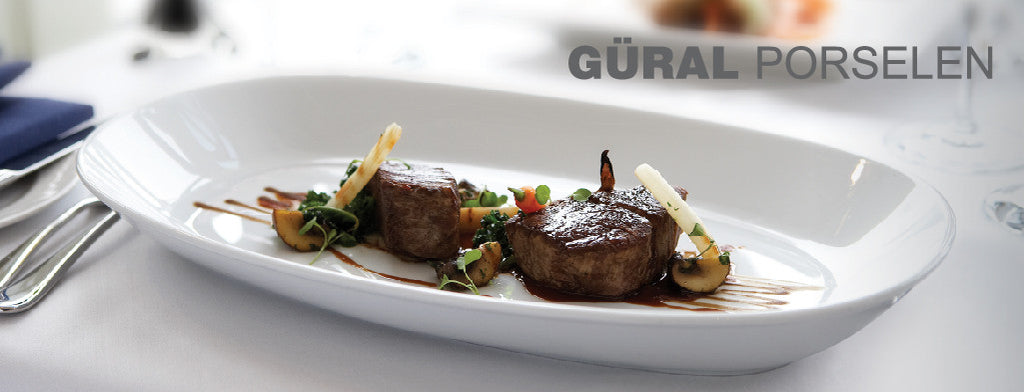Shopping Cart

### Refine

View all

#### Price# Güral

Güral Catalogue£2.86 each (ex VAT)£7.67 each (ex VAT)£1.97 each (ex VAT)£2.46 each (ex VAT)£2.72 each (ex VAT)£2.77 each (ex VAT)£3.08 each (ex VAT)£5.72 each (ex VAT)£7.15 each (ex VAT)£3.62 each (ex VAT)£4.25 each (ex VAT)£2.96 each (ex VAT)£6.96 each (ex VAT)£8.79 each (ex VAT)£3.33 each (ex VAT)£4.26 each (ex VAT)£5.48 each (ex VAT)£7.10 each (ex VAT)£4.02 each (ex VAT)£5.78 each (ex VAT)£7.24 each (ex VAT)£8.95 each (ex VAT)£10.22 each (ex VAT)£14.74 each (ex VAT)£2.95 each (ex VAT)£3.60 each (ex VAT)£6.82 each (ex VAT)£10.37 each (ex VAT)£3.33 each (ex VAT)£4.10 each (ex VAT)£8.34 each (ex VAT)£9.72 each (ex VAT)£5.56 each (ex VAT)£8.09 each (ex VAT)£12.13 each (ex VAT)£10.42 each (ex VAT)£13.55 each (ex VAT)£5.56 each (ex VAT)£10.14 each (ex VAT)£13.98 each (ex VAT)£16.21 each (ex VAT)£18.66 each (ex VAT)£3.18 each (ex VAT)£3.38 each (ex VAT)£4.54 each (ex VAT)£5.93 each (ex VAT)£7.58 each (ex VAT)£9.02 each (ex VAT)